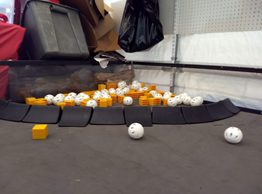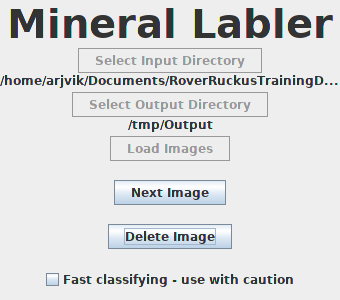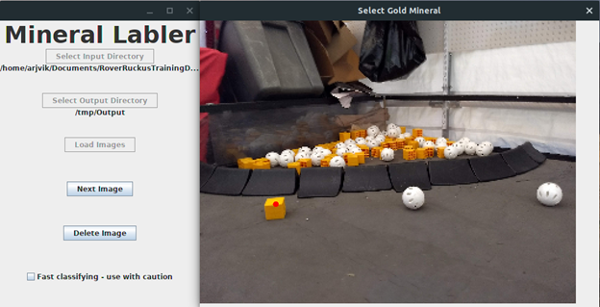Articles by tag: vision

# Vision Discussion

Vision Discussion By Arjun and Abhi

### Task: Consider potential vision approaches for sampling

Part of this year’s game requires us to be able to detect the location of minerals on the field. The main use for this is in sampling. During autonomous, we need to move only the gold mineral, without touching the silver minerals in order to earn points for sampling. There are a few ways we could be able to detect the location of the gold mineral.

First, we could possibly use OpenCV to run transformations on the image that the camera sees. We would have to design an OpenCV pipeline which identifies yellow blobs, filters out those that aren’t minerals, and finds the centers of the blobs which are minerals. This is most likely the approach that many teams will use. The benefit of this approach is that it will be easy enough to write. However, it may not work in different lighting conditions that were not tested during the designing of the OpenCV pipeline.

Another approach is to use Convolutional Neural Networks (CNNs) to identify the location of the gold mineral. Convolutional Neural Networks are a class of machine learning algorithms that “learn” to find patterns in images by looking at large amounts of samples. In order to develop a CNN to identify minerals, we must take lots of photos of the sampling arrangement in different arrangements (and lighting conditions), and then manually label them. Then, the algorithm will “learn” how to differentiate gold minerals from other objects on the field. A CNN should be able to work in many different lighting conditions, however, it is also more difficult to write.

### Next Steps

As of now, Iron Reign is going to attempt both methods of classification and compare their performance.

# CNN Training

CNN Training By Arjun and Abhi

### Task: Capture training data for a Convolutional Neural NetworkIn order to train a Convolutional Neural Network, we need a whole bunch of training images. So we got out into the field, and took 125 photos of the sampling setup in different positions and angles. Our next step is to label the gold minerals in all of these photos, so that we can train a Convolutional Neural Network to label the gold minerals by learning from the patterns of the training data.

### Next Steps

Next, we will go through and designate gold minerals. In addition, we must create a program to process these.

# CNN Training Program

CNN Training Program By Arjun and Abhi

### Task: Designing a program to label training data for our Convolutional Neural NetworkIn order to use the captured training data, we need to label it by identifying the location of the gold mineral in it. We also need to normalize it by resizing the training images to a constant size, (320x240 pixels). While we could do this by hand, it would be a pain to do so. We would have to resize each individual picture, and then identify the coordinates of the center of the gold mineral, then create a file to store the resized image and coordinates.

Instead of doing this, we decided to write a program to do this for us. That way, we could just click on the gold mineral on the screen, and the program would do the resizing and coordinate-finding for us. Thus, the process of labeling the images will be much easier.

Throughout the weekend, I worked on this program. The end result is shown above.

### Next Steps

Now that the program has been developed, we need to actually use it to label the training images we have. Then, we can train the Convolutional Neural Network.

# Labelling Minerals - CNN

Labelling Minerals - CNN By Arjun and Abhi

### Task: Label training images to train a Neural NetworkNow that we have software to make labeling the training data easier, we have to actually use it to label the training images. Abhi and I split up our training data into two halves, and we each labeled one half. Then, when we had completed the labeling, we recombined the images. The images we labeled are publicly available at https://github.com/arjvik/RoverRuckusTrainingData.

### Next Steps

We need to actually write a Convolutional Neural Network using the training data we collected.

# Developing a CNN

Developing a CNN By Arjun and Abhi

### Task: Begin developing a Convolutional Neural Network using TensorFlow and Python

Now that we have gathered and labeled our training data, we began writing our Convolutional Neural Network. Since Abhi had used Python and TensorFlow to write a neural network in the past during his visit to MIT over the summer, we decided to do the same now.

After running our model, however, we noticed that it was not very accurate. Though we knew that was due to a bad choice of layer structure or hyperparameters, we were not able to determine the exact cause. (Hyperparameters are special parameters that need to be just right for the neural network to do well. If they are off, the neural network will not work well.) We fiddled with many of the hyperparameters and layer structure options, but were unable to fix the inaccuracy levels.

 ``` 1 2 3 4 5 6 7 8 9 10 11``` ```model = Sequential() model.add(Conv2D(64, activation="relu", input_shape=(n_rows, n_cols, 1), kernel_size=(3,3))) model.add(Conv2D(32, activation="relu", kernel_size=(3,3))) model.add(MaxPooling2D(pool_size=(8, 8), padding="same")) model.add(Conv2D(8, activation="tanh", kernel_size=(3,3))) model.add(MaxPooling2D(pool_size=(8, 8), padding="same")) model.add(Conv2D(4, activation="relu", kernel_size=(3,3))) model.add(Conv2D(4, activation="tanh", kernel_size=(1,1))) model.add(Flatten()) model.add(Dense(2, activation="linear")) model.summary() ```

### Next Steps

We have not fully given up, though. We plan to keep attempting to improve the accuracy of our neural network model.

# Rewriting CNN

Rewriting CNN By Arjun and Abhi

### Task: Begin rewriting the Convolutional Neural Network using Java and DL4J

While we were using Python and TensorFlow to train our convolutional neural network, we decided to attempt writing this in Java, as the code for our robot is entirely in Java, and before we can use our neural network, it must be written in Java.

We also decided to try using DL4J, a competing library to TensorFlow, to write our neural network, to determine if it was easier to write a neural network using DL4J or TensorFlow. We found that both DL4J and TensorFlow were similarly easy to use, and while each had a different style, code written using both were equally easy to read and maintain.

 ``` 1 2 3 4 5 6 7 8 9 10 11 12 13 14 15 16 17 18 19 20 21 22 23 24 25 26 27 28 29 30 31 32 33 34 35 36 37 38 39 40 41``` ```java //Download dataset DataDownloader downloader = new DataDownloader(); File rootDir = downloader.downloadFilesFromGit("https://github.com/arjvik/RoverRuckusTrainingData.git", "data/RoverRuckusTrainingData", "TrainingData"); //Read in dataset DataSetIterator iterator = new CustomDataSetIterator(rootDir, 1); //Normalization DataNormalization scaler = new ImagePreProcessingScaler(0, 1); scaler.fit(iterator); iterator.setPreProcessor(scaler); //Read in test dataset DataSetIterator testIterator = new CustomDataSetIterator(new File(rootDir, "Test"), 1); //Test Normalization DataNormalization testScaler = new ImagePreProcessingScaler(0, 1); testScaler.fit(testIterator); testIterator.setPreProcessor(testScaler); //Layer Configuration MultiLayerConfiguration conf = new NeuralNetConfiguration.Builder() .seed(SEED) .l2(0.005) .weightInit(WeightInit.XAVIER) .list() .layer(0, new ConvolutionLayer.Builder() .nIn(1) .kernelSize(3, 3) .stride(1, 1) .activation(Activation.RELU) .build()) .layer(1, new ConvolutionLayer.Builder() .nIn(1) .kernelSize(3, 3) .stride(1, 1) .activation(Activation.RELU) .build()) /* ...more layer code... */ .build(); ```

### Next Steps

We still need to attempt to to fix the inaccuracy in the predictions made by our neural network.